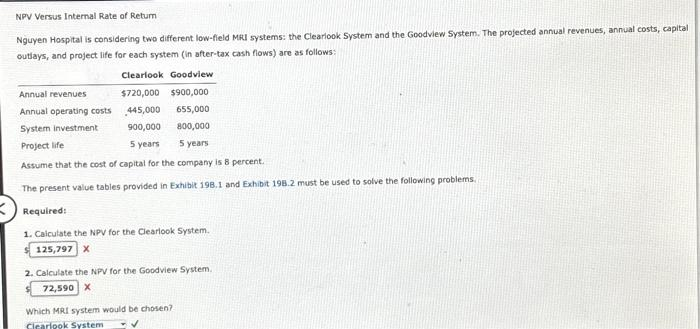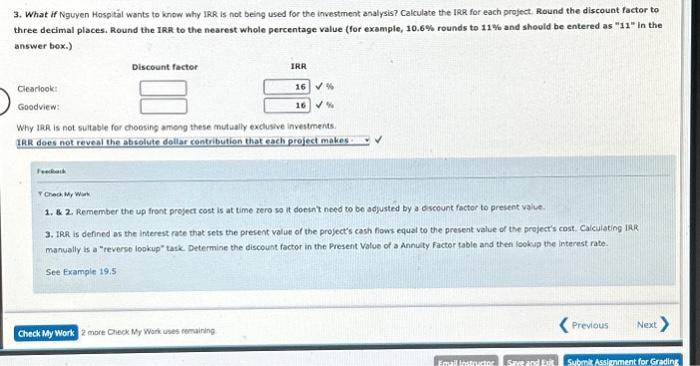Home / Expert Answers / Accounting / npv-versus-internal-rate-of-retum-nguyen-hospital-is-considering-two-different-low-field-mru-system-pa249

# (Solved): NPV Versus Internal Rate of Retum Nguyen Hospital is considering two different low-field Mru system ...NPV Versus Internal Rate of Retum Nguyen Hospital is considering two different low-field Mru systems: the Clearlook System and the Goodview System. The projected annual revenues, annual costs, capital outlays, and project life for each system (in after-tax cash flows) are as follows: Assume that the cost of capital for the company is 8 percent. The present value tables provided in Exhibit 198.1 and Exhibit 198.2 must be used to solve the following problems. Required: 1. Calculate the NPV for the Clearlook System. s 2. Calculate the NPV for the Goodview System. Which MRI system would be chosen? 3. What if Nguyen Hospital wants to know why IRR is not being used for the investment analysis? Calculate the IRR for each project. Round the discount factor to three decimal places, Round the IRR to the nearest whole percentage value (for example, rounds to and should be entered as " 11 " in the answer box.) Why IRR is not sultable for choosing among these mutually exclusive investments. IAR does not reveal the absolute dollar contribution that each project makes: : feckeik F Cheok, Mr Wirk 1. \& 2. Remember the up front project cost is at time zero so it doesnt need to be adjusted by a dscount factor to present value. 3. IRR is defined as the interest rate that sets the present value of the project's cash fiows equal to the present value of the project's cost. Calculating iRR manually is a "reverse lopkup" task. Determine the discount factor in the Present value of a Annuity factor table and then lookip the interest rate. See Example 19.5

We have an Answer from Expert